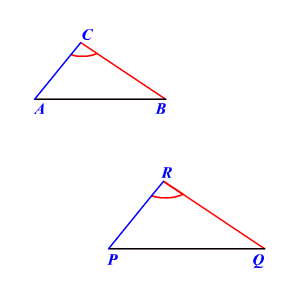# Side-Angle-Side Similarity

If an angle of a triangle is congruent to an angle of another triangle and if the included sides of these angles are proportional, then the two triangles are similar .If $\angle C\cong \angle R\text{\hspace{0.17em}}\text{\hspace{0.17em}}\text{and}\text{\hspace{0.17em}}\text{\hspace{0.17em}}\frac{BC}{QR}=\frac{AC}{PR}$ ,

then the triangles $\Delta ABC\text{\hspace{0.17em}}\text{\hspace{0.17em}}\text{and}\text{\hspace{0.17em}}\text{\hspace{0.17em}}\Delta PQR$ are similar.